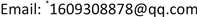﻿ 新疆各城市综合经济发展实力评价——基于因子分析 Factor-Based Analysis of Comprehensive Economic Strength of Cities in Xinjiang Uygur Autonomous Region

World Economic Research
Vol. 07  No. 03 ( 2018 ), Article ID: 26641 , 7 pages
10.12677/WER.2018.73009

Factor-Based Analysis of Comprehensive Economic Strength of Cities in Xinjiang Uygur Autonomous Region

Yazhen Wang, Nannan Fan, Xing Chen*

College of Applied Mathematics, Xinjiang University of Finance and Economics, Urumqi XinjiangReceived: Aug. 6th, 2018; accepted: Aug. 22nd, 2018; published: Aug. 29th, 2018ABSTRACT

Based on the factor analysis method, we studied the comprehensive economic strength of the Xinjiang Uygur Autonomous Region’s cities. According to the characteristics of the Xinjiang Uygur Autonomous Region’s economy, we put forward 10 indicators reflecting the comprehensive economic strength of the city. The research results reflect the differences and imbalances in the economic development of each city, and provide a reference for the development of the Xinjiang Uygur Autonomous Region’s economy.

Keywords:Factor Analysis, Comprehensive Economic Strength, Index System, Evaluation of Results1. 引言

2. 新疆维吾尔自治区各城市经济发展水平的评价

2.1. 建立指标体系

2.2. 因子分析的数据结果与分析

${F}_{1}=0.287{X}_{1}+0.052{X}_{2}+0.178{X}_{3}-0.043{X}_{4}-0.054{X}_{5}+0.288{X}_{6}-0.104{X}_{7}+0.238{X}_{8}+0.275{X}_{9}+0.017{X}_{10}$

${F}_{2}=-0.042{X}_{1}+0.252{X}_{2}-0.278{X}_{3}+0.301{X}_{4}-0.099{X}_{5}-0.067{X}_{6}+0.33{X}_{7}+0.06{X}_{8}-0.092{X}_{9}-0.046{X}_{10}$

${F}_{3}=-0.099{X}_{1}-0.126{X}_{2}-0.533{X}_{3}-0.066{X}_{4}+0.388{X}_{5}-0.083{X}_{6}+0.202{X}_{7}-0.083{X}_{8}-0.043{X}_{9}+0.369{X}_{10}$

$\text{F}=\frac{0.38988{F}_{1}+0.30862{F}_{2}+0.18834{F}_{3}}{0.88683}$Table 1. KMO value and Bartlet test of sample adequacyTable 2. Characteristic values and variance contribution tableTable 3. Factor loading matrix and factor scoring coefficient matrix before and after rotationTable 4. Ranking of comprehensive economic strength of all cities in Xinjiang

3. 结论与建议

3.1. 结论

3.2. 建议

Factor-Based Analysis of Comprehensive Economic Strength of Cities in Xinjiang Uygur Autonomous Region[J]. 世界经济探索, 2018, 07(03): 76-82. https://doi.org/10.12677/WER.2018.73009

1. 1. 廖为鲲, 蔡国梁, 涂文桃. 基于因子分析法的城市经济发展评价[J]. 统计与决策, 2005(24): 52-54.

2. 2. 李莉, 汤银英. 因子分析法在乌鲁木齐城市物流能力评价中的应用[J]. 新疆农业大学学报, 2008, 31(4): 95-98.

3. 3. 刘春云. 新疆经济发展现状及对策[J]. 合作经济与科技, 2010.

4. 4. 刘世薇, 张平宇. 1989-2010年新疆经济发展差异的区域分析[J]. 经济地理, 2012, 32(9): 26-31.

5. 5. 刘晓婷, 陈闻君. 2004-2013年新疆县域经济空间差异演化[J]. 中国沙漠, 2015, 35(4): 1089-1095.

6. 6. 新疆统计局. 新疆统计年鉴[M]. 北京: 中国统计出版社, 2016.

NOTES

*通讯作者。